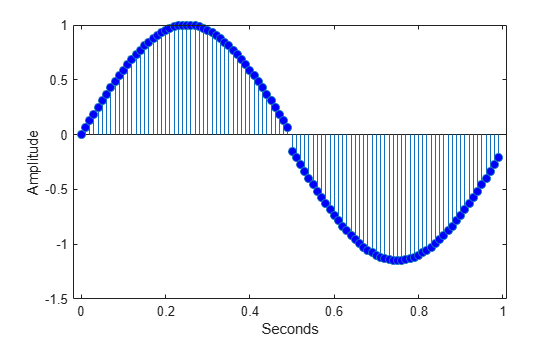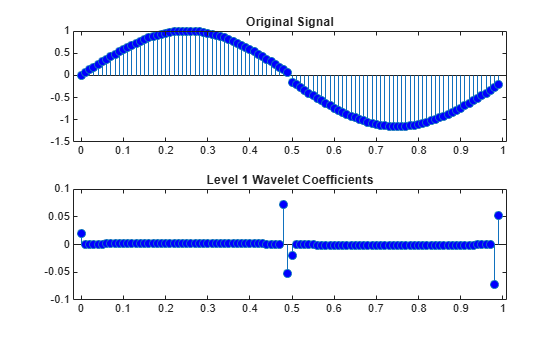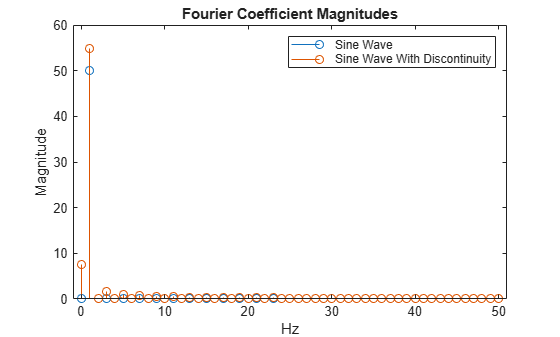## What is a Wavelet?

A wavelet is a waveform of effectively limited duration that has an average value of zero and nonzero norm.

Many signals and images of interest exhibit piecewise smooth behavior punctuated by transients. Speech signals are characterized by short bursts encoding consonants followed by steady-state oscillations indicative of vowels. Natural images have edges. Financial time series exhibit transient behavior, which characterize rapid upturns and downturns in economic conditions. Unlike the Fourier basis, wavelet bases are adept at sparsely representing piecewise regular signals and images, which include transient behavior.

Compare wavelets with sine waves, which are the basis of Fourier analysis. Sinusoids do not have limited duration — they extend from minus to plus infinity. While sinusoids are smooth and predictable, wavelets tend to be irregular and asymmetric.Fourier analysis consists of breaking up a signal into sine waves of various frequencies. Similarly, wavelet analysis is the breaking up of a signal into shifted and scaled versions of the original (or mother) wavelet.

Just looking at pictures of wavelets and sine waves, you can see intuitively that signals with sharp changes might be better analyzed with an irregular wavelet than with a smooth sinusoid.

It also makes sense that local features can be described better with wavelets that have local extent. The following example illustrates this for a simple signal consisting of a sine wave with a discontinuity.

### Localize Discontinuity in Sine Wave

This example shows wavelet analysis can localize a discontinuity in a sine wave.

Create a 1-Hz sine wave sampled at 100 Hz. The duration of the sine wave is one second. The sine wave has a discontinuity at $t=0.5$ seconds.

```t = linspace(0,1,100)'; x = sin(2*pi*t); x1 = x-0.15; y = zeros(size(x)); y(1:length(y)/2) = x(1:length(y)/2); y(length(y)/2+1:end) = x1(length(y)/2+1:end); stem(t,y,'markerfacecolor',[0 0 1]); xlabel('Seconds'); ylabel('Amplitude');```Obtain the nondecimated discrete wavelet transform of the sine wave using the `'sym2'` wavelet and plot the wavelet (detail) coefficients along with the original signal.

```[swa,swd] = swt(y,1,'sym2'); subplot(211) stem(t,y,'markerfacecolor',[0 0 1]); title('Original Signal'); subplot(212) stem(t,swd,'markerfacecolor',[0 0 1]); title('Level 1 Wavelet Coefficients');```Compare the Fourier coefficient magnitudes for the 1-Hz sine wave with and without the discontinuity.

```dftsig = fft([x y]); dftsig = dftsig(1:length(y)/2+1,:); df = 100/length(y); freq = 0:df:50; stem(freq,abs(dftsig)); xlabel('Hz'); ylabel('Magnitude'); legend('sine wave','sine wave with discontinuity');```There is minimal difference in the magnitudes of the Fourier coefficients. Because the discrete Fourier basis vectors have support over the entire time interval, the discrete Fourier transform does not detect the discontinuity as efficiently as the wavelet transform.

Compare the level 1 wavelet coefficients for the sine wave with and without the discontinuity.

```[swax,swdx] = swt(x,1,'sym2'); subplot(211) stem(t,swd); title('Sine Wave with Discontinuity (Wavelet Coefficients)'); subplot(212) stem(t,swdx); title('Sine Wave (Wavelet Coefficients)');```The wavelet coefficients of the two signals demonstrate a significant difference. Wavelet analysis is often capable of revealing characteristics of a signal or image that other analysis techniques miss, like trends, breakdown points, discontinuities in higher derivatives, and self-similarity. Furthermore, because wavelets provide a different view of data than those presented by Fourier techniques, wavelet analysis can often significantly compress or denoise a signal without appreciable degradation.